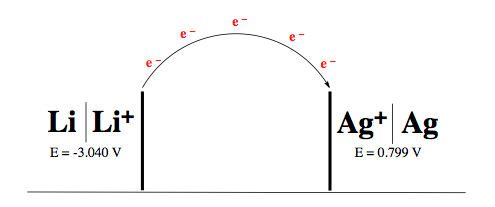Ch.13 - Fundamentals of ElectrochemistryWorksheetSee all chapters
 Ch.1 - Chemical Measurements 2hrs & 10mins 0% complete Worksheet Ch.2 - Tools of the Trade 1hr & 26mins 0% complete Worksheet Ch.3 - Experimental Error 1hr & 51mins 0% complete Worksheet Ch.4 + 5 - Statistics, Quality Assurance and Calibration Methods 2hrs & 1min 0% complete Worksheet Ch.6 - Chemical Equilibrium 3hrs & 43mins 0% complete Worksheet Ch.7 - Activity and the Systematic Treatment of Equilibrium 1hr & 1min 0% complete Worksheet Ch.8 - Monoprotic Acid-Base Equilibria 1hr & 52mins 0% complete Worksheet Ch.9 - Polyprotic Acid-Base Equilibria 2hrs & 16mins 0% complete Worksheet Ch.10 - Acid-Base Titrations 2hrs & 22mins 0% complete Worksheet Ch.11 - EDTA Titrations 1hr & 37mins 0% complete Worksheet Ch.12 - Advanced Topics in Equilibrium 1hr & 18mins 0% complete Worksheet Ch.13 - Fundamentals of Electrochemistry 2hrs & 27mins 0% complete Worksheet Ch.14 - Electrodes and Potentiometry 55mins 0% complete Worksheet Ch.15 - Redox Titrations 1hr & 14mins 0% complete Worksheet Ch.16 - Electroanalytical Techniques 1hr & 23mins 0% complete Worksheet Ch.17 - Fundamentals of Spectrophotometry 55mins 0% complete Worksheet BONUS: Chemical Kinetics Not available yet

Standard Potentials

See all sections
Sections
Basic Concepts
Electrochemical Cells
Standard Potentials
Nernst Equation
Standard Cell Potential & the Equilibrium Constant
Calculating Standard Potential

Concept #1: Voltage (E) represents the amount of work done in an electrochemical cell as electrons travel from one electrode to another.

Example #1: Determine the electric potential that results from the given galvanic cell.Standard Potential Calculations

Example #2: Use the standard half-cell potentials listed below to calculate the standard cell potential for the following reaction occurring in an electrochemical cell at 25°C. Assume the concentrations have approached unity.

3 Cl (g) + 2 Fe (s) ⇌ 6 Cl (aq) + 2 Fe3+ (aq)

Half Reactions:

Cl2 (g) + 2 e  → 2 Cl (aq)                      E° = + 1.396 V
Fe3+ (aq) + 3 e  →  Fe (s)                         E° = – 0.040 V

Example #3: For the a voltaic cell with the overall reaction:

Zn (s) + Cu2+ (aq) ⇌ Zn2+ (aq) + Cu (s)               E°cell = 1.10 V

Given that the standard reduction potential of Zn2+ to Zn (s) is – 0.762 V, calculate the standard reduction potential for:

Cu2+ (aq) + 2 e  →  Cu (s)